# Finding Relative Extrema of a Function: Practice Problems & Explanation

Instructor: Shaun Ault

Shaun is currently an Assistant Professor of Mathematics at Valdosta State University as well as an independent private tutor.

Finding the points at which a function attains its highest or lowest value has many practical applications. In this lesson, you will learn how to use basic calculus to find the relative minimums and maximums of any function. Only basic knowledge of finding derivatives and solving equations using algebra will be necessary to understand this topic.

## Introduction

Where is the best pizza? Depends on who you ask, right? When I think of the best pizza, I'm really just thinking of the best in my city or neighborhood. Let's say it's 'Bob's Pizza.' So Bob's might have the best pizza out of all pizza places near me, but not the best pizza on the planet. Relative to my location, Bob's pizza is a maximum.

## Definitions

A relative maximum is a point at which a function attains its highest value relative to the points nearby. Visually, this occurs at any 'peak' on the function's graph.

Similarly, a relative minimum is a point at which a function attains its lowest value relative to points nearby.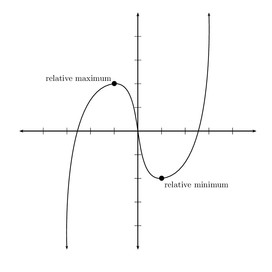Together, relative maxima (plural of maximum) and minima (plural of minimum) are known as the relative extrema of the function. (All those weird words are actually in Latin, but don't worry, we won't be conjugating any verbs today!) A given function could have many extrema. Any peak or valley in the graph counts.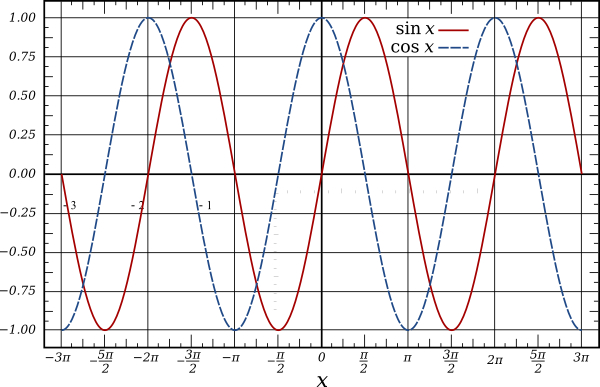As you can probably imagine, these kinds of points are of interest in a variety of settings. For example, if your company has a function that models its profit based on how many units it produces, you would certainly be interested in that function's highest points!

## Finding Relative Extrema

The key to finding these points is this fact: if f(x) is a nice smooth function (no breaks or corners), then at any maximum or minimum (extremum), when the graph is turning around, there must be a horizontal tangent at that point. And this means that the derivative value will be zero at that point, because a horizontal tangent has slope equal to 0.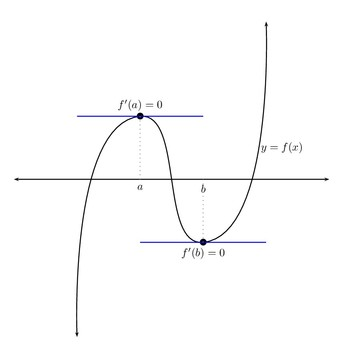## Example 1

Let's do an example: find the relative maximum and minimum of the function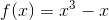This is the function whose graph is pictured near the top of this article. The 'maximum' or 'minimum' feature under the CALC menu on your trusty Texas Instruments calculator would tell you that the maximum and minimum occur around x=-0.577 and x=0.577 respectively. However, our skills in finding derivatives will help us find exactly where these points lie. There is an easy 3-step method.

• Step 1: Find the derivative of f.

Remembering the power rule for derivatives, we find that: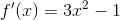To unlock this lesson you must be a Study.com Member.

### Register to view this lesson

Are you a student or a teacher?